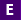#Not a Florida public school educator?

Access this resource
on CPALMS.com

### General Information

Subject(s): Mathematics
Intended Audience: EducatorsSuggested Technology: Internet Connection
Instructional Time: 40 Minute(s)
Keywords: data, data collection, data analysis, graph, computer science, math, computer, statistics, quantitative, quantitative data, qualitative, qualitative data, list, programming, index, data types, if/then statements, variables, local variables, global variables, if/then else statements, x-axis, y-axis, range, app, programming
Instructional Component Type(s):

## Aligned Standards

This vetted resource aligns to concepts or skills in these benchmarks.

## 1 Lesson Plan

Gather Data For Distribution by Programming an App

This lesson allow students to gather, calculate, and plot data using both computer code and mathematical equations. In this lesson students will create a pedometer app to demonstrate the understanding of algorithms, components (such as buttons, textboxes, sensors, etc.), and If/Then statements. This lesson uses algebraic equations and random data to access the needed components to store data in a spreadsheet.

## Related Resources

Other vetted resources related to this resource.

## Lesson Plan

Gather Data For Distribution by Programming an App:

This lesson allow students to gather, calculate, and plot data using both computer code and mathematical equations. In this lesson students will create a pedometer app to demonstrate the understanding of algorithms, components (such as buttons, textboxes, sensors, etc.), and If/Then statements. This lesson uses algebraic equations and random data to access the needed components to store data in a spreadsheet.

Type: Lesson Plan